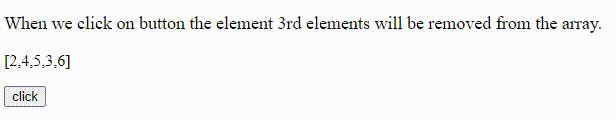Open In App

# How to remove specific elements from the left of a given array of elements using JavaScript ?

In this article, we will learn How to remove specific elements from the left of a given array of elements using JavaScript. We have given an array of elements, and we have to remove specific elements from the left of a given array.

Approach: The splice() method is used to add and remove elements from an array. To remove specific elements from the left of a given array, we will use the splice method. To remove specific elements it takes two parameters index form where we want to remove the element and the number of the elements we want to remove. It returns an array that contains removed elements.

Syntax:

`array.splice(index,No_of_element);`

Example: In this example, we will remove specific elements from the left of a given array of elements using JavaScript using the splice() method.

## HTML

 `<``body``>``    ``<``div``>``        ``<``p` `id``=``"p"` `style``=``"font-size: 18px"``>``            ``When we click on button the element``            ``3rd elements will be removed from``            ``the array.``        ```` ` `        ``<``p` `id``=``"gfg"``>[2,4,5,3,6]`` ` `        ``<``button` `onclick``=``"fun(5)"``>click``    ```` ` `    ``<``script``>``        ``function fun(n) {``          ``// Array``          ``var arr = [2, 4, 5, 3, 6];``           ` `          ``// Find index of specified element which is n``          ``var ind = arr.indexOf(n);``           ` `          ``// And remove n from array``          ``arr.splice(ind, 1);``          ``document.getElementById("p").innerHTML = ``          ``"After remove element";``           ` `          ``// Final result after remove n from array``          ``document.getElementById("gfg").innerHTML = ``          ``"[" + arr + "]";``        ``}``    `````

Output: